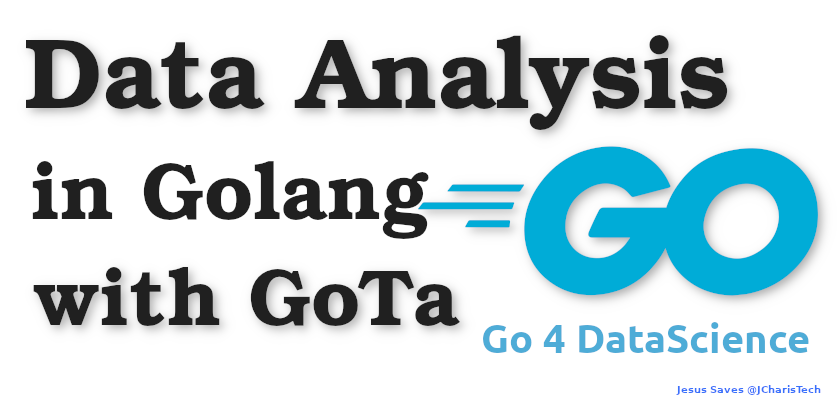# Data Analysis with Golang

In the age of Internet and AI , data is quite important. As the volumne of data increase we will need performant systems to be able to analyse them.
In this tutorial we will explore how to use Golang to Analysis Data.Python is mostly used for data science and data analysis – but let us see how to use Go/Golang for data analysis.

By the end of this tutorial we will learn about

• Go Packages for Data Analysis
• How to Read CSV Dataset in Golang
• Basic Exploratory Data Analysis in Go
• etc

#### GO Packages For Data Analysis

As we learnt in a previous post – performing data cleaning and data exploration in Go is time consuming and not as easy and simple like Python,Julia and R.
However we it is still doable? Let us check the various packages we can use for data analysis

• GoTa
• qFrames
• Dataframes-go
• Gonum
• Stat

Most of these packages are quite useful and have their benefits. Let us pick one of them GoTa and see how to use if for data analysis.

#### Installing GoTa

To install gota you can use the go get command and the link to the package. You can also search for the package on pkd.go.dev

go get github.com/kniren/gota/dataframe

In certain cases you can work with go mod approach to keep track of the packages you will be using in your projects but let us install is globally.

#### How to Read CSV Files

To be able to read csv files you will have to first open the file and then parse and process it using gota/dataframe package. You can also parse it with any of the packages listed above after opening the CSV files.

Opening the CSV Files
There are several ways to open a CSV File in Golang. These include using the Os Package or the CSV package.

We will be analysing the diamonds dataset which you can get from here or on UCI Machine learning repository.

```package main
import (
"fmt"
"log"
"os"
`"github.com/go-gota/gota/dataframe"`
)
func main() {
// Open CSV
csvfile, err := os.Open("data/diamonds.csv")
if err != nil {
log.Fatal(err)
}
`// Read CSV `
`df := dataframe.ReadCSV(csvfile) `
`fmt.Println(df) `
}```

We can now run the program with go run main.go

We can also check out the shape, the number of row and cols as well as the column names using the following

```// Shape of Dataset
row, col := df.Dims()
fmt.Println("Shape of DF:", row, col)

// Get Only Row Size
fmt.Println(df.Nrow())

`// Get only Columns Size `
`fmt.Println(df.Ncol()) `

`// Get Column Names `
`fmt.Println(df.Names())`
```

We can also check out the descriptive stati/summary as well as the datatypes using the following

`// Get DataTypes// fmt.Println(df.Types())`
``````// Describe/Summary
// fmt.Println("Summary", df.Describe())``````

One important thing when performing data analysis is on selection of columns and rows.

``````// Select  columns by Column name
fmt.Println(df.Select("carat"))

// Select column by index
fmt.Println(df.Select(1))``````

You can also select multiple columns.

``````// Multiple Columns Selection
// df[["carat","cut"]]
fmt.Println(df.Select([]string{"carat", "cut"}))``````

#### Selection of Rows

For selection of rows you can use the .Subset() function

``````// Selection of Rows
// Subset : iloc
fmt.Println(df.Subset(0))``````

#### Working with Series

``````// Series and Apply Functions
ds := df.Col("carat") // A Series
fmt.Println(ds)
fmt.Printf("%T \n", ds)``````

#### Applying Functions

``````// Get the mean
dsmean := ds.Mean()
fmt.Println("Mean of Series:", dsmean)

// Get the Mean using Another Package
gmean := stat.Mean(ds.Float(), nil)
fmt.Println("Go Num Mean for series:", gmean)``````

#### Check For Missing Values

`fmt.Println(ds.IsNaN())`

#### Working with Conditions using Filter

``````fmt.Println(df.Select("cut"))